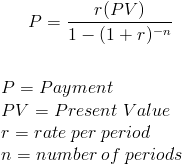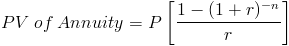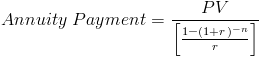# Annuity Payment (PV)The annuity payment formula is used to calculate the periodic payment on an annuity. An annuity is a series of periodic payments that are received at a future date. The present value portion of the formula is the initial payout, with an example being the original payout on an amortized loan.

The annuity payment formula shown is for ordinary annuities. This formula assumes that the rate does not change, the payments stay the same, and that the first payment is one period away. An annuity that grows at a proportionate rate would use the growing annuity payment formula. Otherwise, an annuity that changes the payment and/or rate would need to be adjusted for each change. An annuity that has its first payment due at the beginning would use the annuity due payment formula and the deferred annuity payment formula would have a payment due at a later date.

The annuity payment formula can be used for amortized loans, income annuities, structured settlements, lottery payouts(see annuity due payment formula if first payment starts immediately), and any other type of constant periodic payments.

## Per Period

The rate per period and number of periods should reflect how often the payment is made. For example, if the payment is monthly, then the monthly rate should be used. Likewise, the number of periods should be the number of months. This concept is important to remember with all financial formulas.

## Annuity Payment Formula Explained

The annuity payment formula can be determined by rearranging the PV of annuity formula.After rearranging the formula to solve for P, the formula would become:This can be further simplified by multiplying the numerator times the reciprocal of the denominator, which is the formula shown at the top of the page.

New to Finance?